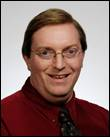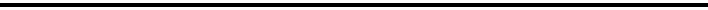Dr. Charles Patterson

Instructor of Mathematics & Statistics

College of Engineering & Science

Louisiana Tech University

P.O. Box 10348

Ruston, LA 71272-0046

Office Location: Bogard Hall 217A

Office Phone:  (318) 257-3224

FAX:   (318) 257-2182

Email:  charlesp@latech.eduCOURSE INFORMATION (LA Tech Campus):

Math 099: Preparation for College Mathematics

Math 101: College Algebra

Math 102: Applied Algebra for College Students

Math 103: Applied Algebra for College Students

Math 113:  Plane Geometry

Math 203:  Introduction to Number Structure

Math 204: Conceptual Geometry & Quantitative Analysis

Math 301: Functions and Modeling

Math 302: Introduction to Geometry and Mathematical FoundationsINTERNET COURSES:

Math 100: College Algebra (Internet)

Math 101: College Algebra (Internet)

Math 112: Trigonometry (Internet)DUAL ENROLLMENT COURSES:

Math 099: Preparation for College Mathematics (Dual Enrollment)MATH CREDIT EXAM INFORMATION: